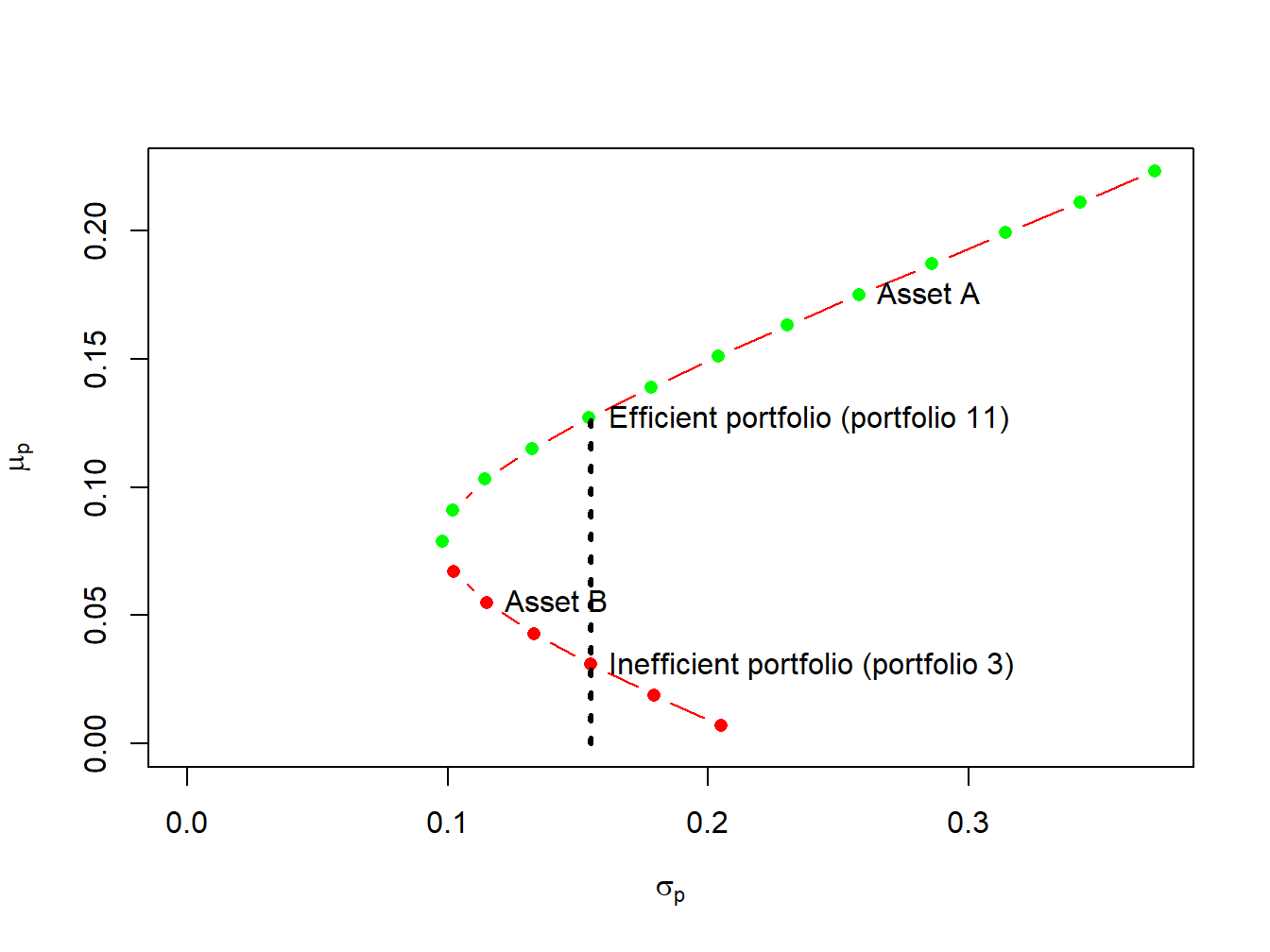## 11.3 Efficient portfolios with two risky assets

Given the assumptions on returns and investor risk preferences, we now characterize the set of efficient portfolios and inefficient portfolios.

Definition 11.1 Efficient portfolios are the feasible portfolios that have the highest expected return for a given level of risk as measured by portfolio standard deviation. These are the portfolios that investors are most interested in holding. Graphically, these are the portfolios starting with the global minimum variance portfolio that are above and to the right of the global minimum variance portfolio.

Definition 11.2 Inefficient portfolios are the portfolios such that there is another feasible portfolio that has the same risk but a higher expected return. Graphically, these are the portfolios that are below and to the right of the global minimum variance portfolio.Figure 11.5: Efficient and inefficient portfolios for example data.

Example 2.12 (Efficient and inefficient portfolios for example data)

Figure 11.5 shows the efficient and inefficient portfolios from the example data in Table 11.1. The efficient portfolios are those that have the highest expected return for a given standard deviation value. These portfolios are the green dots starting with the global minimum variance portfolio at the tip of the Markowitz bullet. The inefficient portfolios are the red dots below the global minimum variance portfolio. For example, Figure 11.5 shows two feasible portfolios, portfolio 3 and portfolio 11, with the same standard deviation value but different expected return values. Portfolio 11 has the highest expected return and so is the efficient portfolio. Portfolio 3, with the lower expected return, is the inefficient portfolio.

$$\blacksquare$$

### 11.3.1 Optimal portfolios

Given the efficient set of portfolios as described in Figure 11.2, which portfolio will an investor choose? Of the efficient portfolios, investors will choose the one that accords with their risk preferences. Very risk averse investors will want a portfolio that has low volatility (risk) and will choose a portfolio very close to the global minimum variance portfolio. In contrast, very risk tolerant investors will ignore volatility and seek portfolios with high expected returns. Hence, these investors will choose portfolios with large amounts of asset $$A$$ which may involve short-selling asset $$B$$.